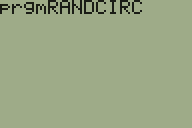Satisfactory TI-84 Plus Programs

A few days ago, I decided to create some programs that are good to spend time. Some of them make you relax and others make you even more stressful. Anyway, I will share some of them with you.

Graphic Reverser

The objective of this program is to reverse all the pixels in the graphic area.

To achieve this, we will need to implement a loop that iterates over all the screen and changes the color of the pixels using PxlChange function.

0→X
0→Y
While X≤94
0→Y
While Y≤62
Pixel-Change(Y,X
(Y+1)→Y
End
(X+1)→X
End

Effect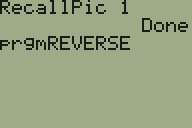Make The Circle Great Again

This program starts by creating a small circle in the middle of the screen and the recursively increases its radius.

To make this, you just need to understand how the Circle function works, taking the coordinates and the radius as input. In the following code, you can change the value 0.5 to output different patterns.

ClrDraw
AxesOff
ZSquare
0.5→X
While X≤18
Circle(0,0,X)
(0.5+X)→X
End

Effect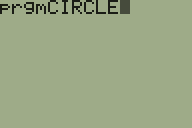Random Stars

This program changes random pixels in the calculator screen to create a random pattern of black points. Once again, the function PxlChange is used in conjunction with an infinitive loop.

ClrDraw
AxesOff
While 1
randInt(0,62)→Y
randInt(0,94)→X
Pxl-Change(Y,X)
End

Effect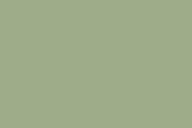Random Lines

For those wondering if I have any program faster than the already mentioned ones, yes, I also created Random Lines.

Code

ClrDraw
ZSquare
AxesOff
While 1
randInt(-­15,15)→Y
randInt(-­10,10)→X
randInt(-­15,15)→A
randInt(-­10,10)→B
Line(Y,X,A,B)
End

Effect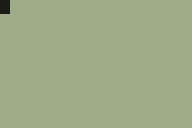Random Circles

And now, the last one, a program that randomly outputs circles to random screen coordinates with random radius.

Code

ClrDraw
ZSquare
AxesOff
While 1
randInt(­-10,10)→X
randInt(­-15,15)→Y
randInt(1,8)/2→R
Circle(Y,X,R)
End

Effect